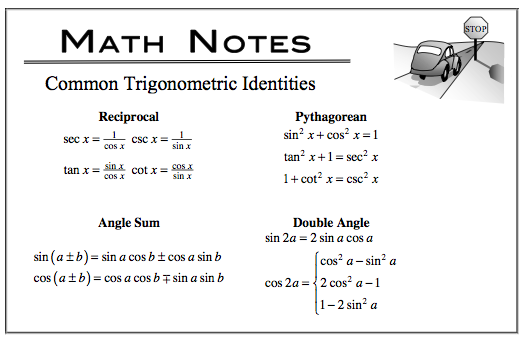### Home > CALC > Chapter 8 > Lesson 8.1.1 > Problem8-8

8-8.
1. No calculators! Evaluate the following integrals. Homework Help ✎1.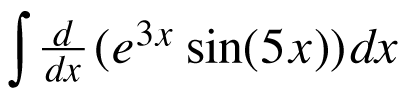2.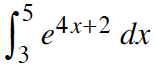3.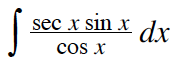4.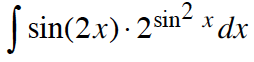5.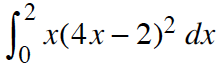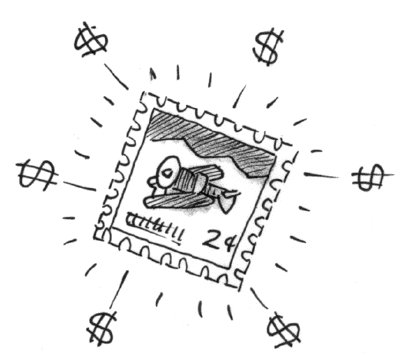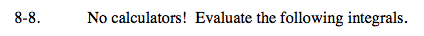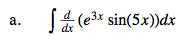Remember that the integral 'undoes' the derivative.But notice that you are integrating as a final step: don't forget +C.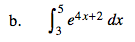Recall that e4x + 2 = e2e4x and e2 is a constant.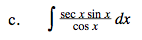$\text{Recall that sec}(x)=\frac{1}{\text{cos}(x)}\text{ and }\text{tan}(x)=\frac{\text{sin}(x)}{\text{cos}(x)}?$

Before integrating, rewrite the integrand so it is not a fraction.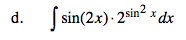Use u-substitution.
u = sin²x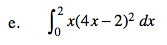Before integrating, expand the integrand.

$=\int_0^2\Big(16x^3-16x^2+4x\Big)dx$